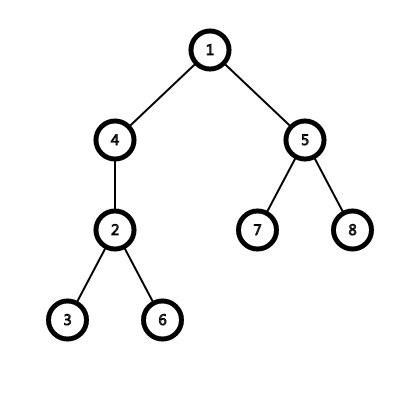# Orange Boy Can You Solve It Out? Ep.7

unsolvable 思考题 for unsolvable monkey

# Dragon Bone Reborn

As a dragon yourself, it is very hard for you to live for every day there're a lot of knights who want to kill you and your bones are frequently broken.
Your bone system can be seen as a undirected tree T with N nodes and M edges. Each edge represents a bone and on each node there is a "repairer" who can repair your bone.
A process of repairing goes as follows:
Assume that the bone connecting (x,y) is broken, you firstly call some of the repairers to rush to node x or y.Note that the repairer cannot pass the broken bone. The time cost of this process is the maximum distance for each repairer to come.
Then they will start repairing after everyone is here. Assume that the "seriousness" of the injury is W and S repairers have come, then the time for this process is W/S rounding up
So the total repairing process time cost is the sum of the two subprocesses.
Now given M situations, in each situation you know what bone is broken and the seriousness. For each situation,print the minimal time needed to repair it.

## Example Input

T={
1 4
1 5
2 3
2 4
5 7
5 8
2 6
}
Query={
[2->4,serious=5],
[1->5,serious=7]
}

2
3

# Example ExplainFor query 1, if we choose {2,4} then cost is 0+5/2=3
if we choose {2,4,1,3,6} then cost is 1+5/5=2
if we choose {5,1,4,2,3,6} then cost is 2+5/6=3
....
it can be proven that answer is 2.
For query 2, if we choose {1,5} then cost is 0+7/2=4
if we choose {4,1,5,7,8} then cost is 1+7/5=3
if we choose {2,4,1,5,7,8} then cost is 2+7/6=4
if we choose {2,4,1,5,7,8,3,6} then cost is 3+7/8=4
....
It can be proven that answer is 3

## Constriants

Subtask2(40%): n,m<=10^5,all seriousness is the same